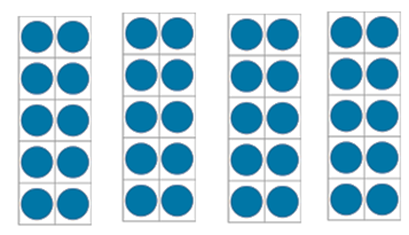Mathematics
Easy

Question

# Count by 10s write the number## 30407920Hint:

## The correct answer is: 40

### Here, we have to count the number by 10s.Total number of figures = 4So, the number = 10+10+10+10 = 40.Hence, the correct option is C.

Multiplication is repetitive addition. We can just multiply 10 by 4.

### Related Questions to study#### With Turito Foundation.#### Get an Expert Advice From Turito.